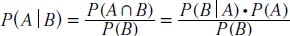NextPrevious

# What is Bayes’s theorem?

Bayes’s theorem is a result that lets new information be used to update the conditional probability of an event. The theorem was first derived by English mathematician Thomas Bayes (1702–1761), who developed the concept to use in situations in which probability can’t be directly calculated. The theorem gives the probability that a certain event has caused an observed outcome by using estimates for all possible outcomes. Its simplest notation is as follows:Close

This is a web preview of the "The Handy Math Answer Book" app. Many features only work on your mobile device. If you like what you see, we hope you will consider buying. Get the App